### 8. INTEGRATED PHOTOMETRY

In order to obtain integrated photometry of the galaxies in our sample we edited the final, flux-calibrated images of the Atlas. Field stars and background galaxies falling near the position of our galaxies were removed using the IRAF task CREDIT within the CRUTIL package. We did this for all the B and R-band images. Emission from field stars and from background galaxies in the Himages was removed at the time of the Hcontinuum subtraction.

The criteria for identifying a region as belonging to the galaxy (or not) were those used by Gil de Paz et al. (2000b). Briefly, these criteria are based (1) on the size and ellipticity of the emitting region compared to the image PSF, (2) the presence of Hemission associated with the region, and (3) the distance of the region from the galaxy center (see Gil de Paz et al. 2000b for more details).

Once all the images were edited we defined two sets of polygonal apertures. The first set was constructed to include the total integrated light originating from the galaxy at continuum wavelengths, and it was identically used to measure both the B and R-band integrated magnitudes. Due to the different spatial distribution and morphology of the Hemission compared with the continuum, the integrated Hfluxes were measured using different sets of polygonal apertures. In both cases, the integrated fluxes were obtained using the IRAF task POLYPHOT.

The color term required to determine the B and R-band magnitudes of the galaxies was first computed assuming a mean (B - R) color of 0.8mag and using the color coefficients given in Table 4. However, the integrated colors derived were in some cases significantly different from this average value. The final magnitudes and colors were then iteratively computed using the limit of the following sequence as the best (B - R) color for the galaxy,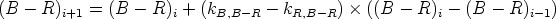(2)

where kB, B-R and kR, B-R are the color coefficients for the B and R bands, respectively. This sequence ranges from i = 1 to n, where (B - R)0 = 0.8 mag and (B - R)1 is the integrated color initially measured on the images. Convergence ((B - R) << 0.01) occurred after a few (n ~ 5-10) iterations. The final magnitudes and colors are given in Table 5. The errors shown in this table were obtained by combining the photometry errors given by the task POLYPHOT with those associated with the calibration of the images. Fluxes, magnitudes and colors shown are corrected for Galactic extinction (using the values given in Table 1 and the Galactic extinction law of Cardelli, Clayton, & Mathis 1989), but they are not corrected for internal extinction. Hfluxes given in Table 5 are also corrected for underlying stellar absorption adopting an equivalent width of -3Å (González-Delgado, Leitherer, & Heckman 1999).

We have compared our integrated magnitudes measured with the asymptotic values given by Doublier et al. (1997, 1999) and Cairós et al. (2001b) and the BT and RT magnitudes in the RC3 and ESO-LV catalogs (BT, de Vaucouleurs et al. 1991; BT, RT; Lauberts & Valentijn 1989). The mean difference between the B-band magnitudes given by Cairós et al. (2001b) and ours for a total of 14 galaxies in common is +0.06 mag with an rms of ± 0.22 mag (our magnitudes are marginally brighter). The comparison between the total B-band magnitudes in the RC3 catalogue and our observed magnitudes gave a difference of -0.04 ± 0.19 mag (44 galaxies in common). The largest B-band difference (+0.36 ± 0.34 mag) is obtained when comparing with the results for 23 galaxies in common with Doublier et al. (1997, 1999). For the R-band data the differences are -0.22 ± 0.20 mag (11 galaxies) and -0.15 ± 0.36 mag (26 galaxies) with respect to the Cairós et al. (2001b) and Doublier et al. (1997, 1999) samples, respectively. This systematic difference is reduced to -0.05 ± 0.16 mag (9 galaxies) when comparison is made with the R-band magnitudes in the ESO-LV catalogue. Note that the comparison with the RC3 and ESO-LV catalogs was done using observed magnitudes (i.e. not corrected for Galactic extinction). Finally, we compared the aperture-photometry data of Cairós et al. (2001a) with our results. This yields differences of +0.16 ± 0.18 mag (14 galaxies) and -0.10 ± 0.23 mag (11 galaxies) in the B and R bands, respectively. The existence of these differences is attributed to (1) intrinsic differences between the extrapolated asymptotic magnitudes and our aperture-photometry data, (2) the different galactic-extinction maps used (Burstein & Heiles 1984 or Schelegel et al. 1998), and (3) the different methods adopted for the removal of field stars and background galaxies falling near the position of the galaxies under study.

Absolute magnitudes and Hluminosities were derived using the distances given in Table 1. We computed the equivalent widths of Hby dividing the Hflux by the flux (per unit wavelength) in the R-band after taking into account the added contribution of Hto the observed R-band magnitude itself (see Appendix A for more details).

In Figures 4a & 4b we show the frequency histograms in (B - R) color and B-band absolute magnitude. Average color and absolute magnitude of the galaxies in our sample are (B - R) = 0.7 ± 0.3 mag and MB = -16.1 ± 1.4 mag. The average Hluminosity is log(LH) = 40.0 ± 0.6 (erg s-1). In Panels 4c, 4d, 4e, and 4f we have plotted, respectively, (B - R) vs. MB, EW(H) vs. MB, LHvs. MB, and EW(H) vs. (B - R), using different symbols for each morphological type (dots, nE; filled-stars, iE; open-squares, iI; open-circles, i0).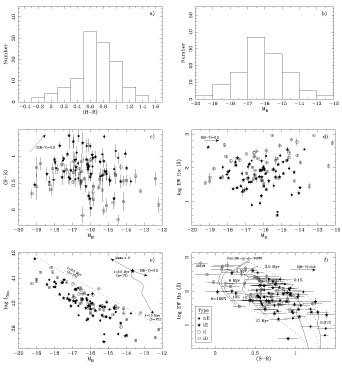Figure 4. a) Frequency histogram of the (B - R) color. b) Frequency histogram of the absolute magnitude, MB. c) Color vs. MB diagram. Different symbols are used for nE, iE, iI, and i0 BCDs (see legend in Panel f). The effect of a color excess of E(B - V) = 0.2 mag is shown by arrows. d) EW(H) vs. MB diagram. e) Hluminosity vs. MB diagram. Dotted-line shows the properties of a composite stellar population formed by a 3.5-Myr-old burst with Z/5 metallicity and 1% burst strength in mass overimposed on a 9-Gyr-old underlying stellar population with the same metallicity. The effects of a change in the age of the burst, the internal extinction and total mass of the galaxy are also shown. f) EW(H) vs. (B - R) color diagram. The predictions of the models for the stellar population described above are shown. Solid lines represent models of constant burst strength and age between 3.5 and 10 Myr. Dotted lines are models with constant age but different burst strength.

Figure 4c shows that fainter BCD galaxies tend to have bluer colors. Also the galaxies classified as iI and i0 BCD show, on average, bluer colors than those in the nE and iE classes. This same difference is also present in the case of the EW(H) (see Figure 4d), where iI and i0 BCDs show significantly larger equivalent widths. The average colors and equivalent widths of the nE and iE BCDs are (B - V) = 0.8 mag and EW(H) = 90Å, respectively, while for the iI and i0 BCDs these values are (B - V) = 0.5 mag and EW(H) = 200Å. If we consider only the galaxies classified as iI BCDs the average colors and EW(H) values derived are 0.6 mag and 150Å, respectively. Moreover, Figure 4c also shows that for (B - R) < 0.5 about 27% (6 over 22) of the galaxies are nE/iE types while for (B - R) > 0.95 this percentage goes up to 95% (18/19). With regard to the equivalent width of H, Figure 4d indicates that for logEW(H) > 2.4 about 23% (3/13) are nE/iE BCDs while for logEW(H) < 1.2 the percentage is 100% (14/14). The lack of objects showing both low continuum and Hluminosity (lower-right corner of Figure 4d) is mainly due to the selection effects associated with the objective-prism surveys searching for emission-line galaxies from whose many galaxies in our sample were selected. In these surveys the probability of detection is mainly driven by the emission-line flux and its contrast against the continuum (Salzer 1989). Therefore, objects with low luminosity will be detected only if the contrast between the line and the continuum is very strong, in other words, if the equivalent width is large ( > 20Å typically).

The dotted-line in Figure 4e represents the model predictions for a composite stellar population formed by a 3.5-Myr-old burst with Z/ 5 metallicity and 1% burst strength in mass superimposed on a 9-Gyr-old underlying stellar population with the same metallicity. The independent effects of a change in the age of the burst, the internal extinction and total mass of the galaxy are also shown. These models were extensively described in Section 3.

Finally, in Figure 4f we show the distribution of our galaxies in the EW(H) vs. (B - R) color diagram along with the predictions of the same models for different values of the burst age and burst strength, ranging from 3.5 to 10 Myr and from 0.01 to 100%, respectively. This figure shows that in most of the BCDs in our sample ( ~ 80%) the presence of an evolved, underlying stellar population is required, even if a moderate internal extinction of E(B - V) = 0.2 mag is assumed and differences in metallicity between individual galaxies are taken into account. This value of the color excess corresponds to the most frequently found value in the spectroscopic atlas of BCDs of Terlevich et al. (1991). The most metal-poor objects in our sample, however, do not appear to require an evolved stellar population to reproduce their (B - R) colors and EW(H). But, the (B - R) color is not very sensitive to the presence of an evolved stellar population when the burst strength is larger than a few percent, so the existence in these galaxies of such an evolved population cannot be ruled out by these data. The combination of optical data and deep near-infrared observations is crucial in solving this problem (James 1994; Doublier et al. 2001b, Vanzi et al. 2002; Noeske et al. 2003, submitted).

Figure 4f also confirms (see above) that there is a clear difference between the properties of the nE/iE BCDs (filled symbols) and those of the iI/i0 BCDs (open symbols). BCD galaxies classified as nE and iE types are significantly redder and show lower EWs in Hthan the iI and i0 BCDs. This is probably due to (1) a lower dust extinction, (2) higher burst strength, and/or (3) lower metallicity of the iI and i0 galaxies compared to the nE and iE BCDs. Some differences in this same sense have been already pointed out by Noeske et al. (2000) for the case of the iI,C (cometary) BCDs.

It is worth noting that, despite the number of surveys involved, different selection criteria, and different physical sizes and environments where these galaxies were discovered, there are observational properties that are common to all BCD galaxies within the same morphological class, although with a significant dispersion. This suggests that the morphology of these galaxies is direct testimony to their merging and star-formation histories.

In order to show the wide range of morphologies and physical sizes spanned by these galaxies, and its relation to their luminosities and optical colors, we have plotted together the R-band and Hmaps for 80 of the galaxies in the sample set to a common distance and using a common surface brightness scale (see Figures 5 and 6). Due to the dense clustering of galaxies at certain positions in the color-magnitude diagram (see Figure 4c) the representation of the complete sample of 114 objects in Figures 5 and 6 is not possible. The R-band and Himages of the galaxies are shown in boxes of fixed physical size of 5 kpc on a side using a gray scale ranging from the sky level (white) to a surface brightness of 21mag/arcsec-2 in R and 1.5 × 10-15 erg s-1 cm-2 arcsec-2 in H(black) (these surface brightness are observed values, except for the highly obscured objects II Zw 40 and IC 10 which were corrected for Galactic extinction). Figure 6 shows that, as commented on above, the largest HEWs are found within those objects showing the lowest luminosities and bluest colors (bottom-right in this figure). These images also graphically illustrate that BCD galaxies cover, at least, one order of magnitude in physical size, from ~ 0.3 kpc to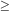3 kpc. A more detailed analysis of the physical size, structure, and population content of BCDs will be carried out in subsequent papers.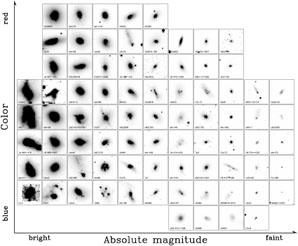Figure 5. R-band images of 80 of the galaxies in the sample. All panels are 5 kpc in size. The grayscale ranges between the value of the sky (white) and that corresponding to a surface brigthness of 21 mag arcsec-2 (black). The name of the galaxy is shown at the bottom-left corner of each panel.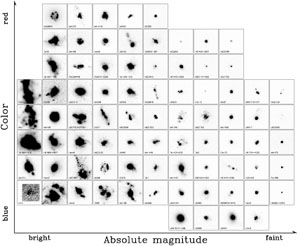Figure 6. Continuum-subtracted Himages of 80 of the galaxies in the sample. All panels are 5 kpc in size. The grayscale ranges between the value of the sky (white) and that corresponding to a surface brigthness of 1.5 × 10-15 erg s-1 cm-2 arcsec-2 (black). The name of the galaxy is shown at the bottom-left corner of each panel.